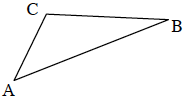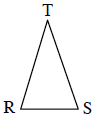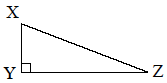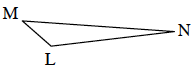### Home > CC2MN > Chapter 4 > Lesson 4.4.2 > Problem4-142

4-142.

If each triangle below is drawn accurately, identify whether it is an obtuse, acute, or right triangle. Name the angle or angles that helped you to decide.

1.Examine all three angles of the triangle.
Is the measure of any of the angles greater than or equal to $90°$?

$m\angle C$ is greater than $90°$, so this figure is an obtuse triangle.

1.See part (a).

Since all the angles measure less than $90°$, the figure is an acute triangle.

1.What does the box on angle Y mean?

The box means that the angle at that point is a right angle.
How can you identify this triangle?

1.See part (a).